Courses

# Points, Lines, Planes and Angles Mathematics Notes | EduRev

## Mathematics : Points, Lines, Planes and Angles Mathematics Notes | EduRev

The document Points, Lines, Planes and Angles Mathematics Notes | EduRev is a part of the Mathematics Course Additional Topics for IIT JAM Mathematics.
All you need of Mathematics at this link: Mathematics

An introduction to geometry
A point in geometry is a location. It has no size i.e. no width, no length and no depth. A point is shown by a dot.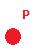A line is defined as a line of points that extends infinitely in two directions. It has one dimension, length. Points that are on the same line are called collinear points.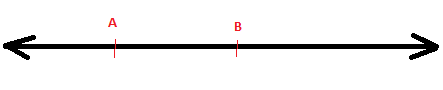A line is defined by two points and is written as shown below with an arrowhead.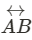Two lines that meet in a point are called intersecting lines.
A part of a line that has defined endpoints is called a line segment.  A line segment as the segment between A and B above is written as: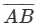A plane extends infinitely in two dimensions. It has no thickness. An example of a plane is a coordinate plane. A plane is named by three points in the plane that are not on the same line. Here below we see the plane ABC.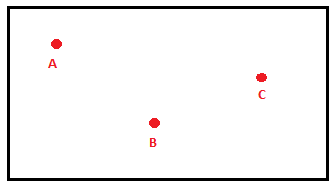A space extends infinitely in all directions and is a set of all points in three dimensions. You can think of a space as the inside of a box.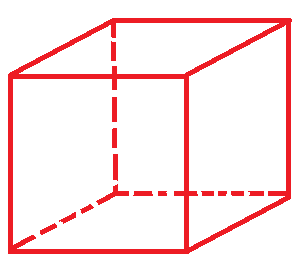Measure line segments
The length of a line segment can be measured (unlike a line) because it has two endpoints.  As we have learnt previously the line segment can be written as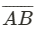While the length or the measure is simply written AB. The length could either be determined in Metric units (e.g. millimeters, centimeters or meters) or Customary units (e.g. inches or foot).
Two lines could have the same measure but still not be identical.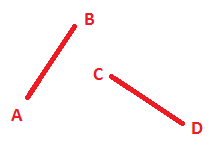AB and CD have the exact same measure and are said to be congruent and is noted as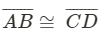This is read as the line AB is congruent to the line CD.

Finding distances and midpoints
If we want to find the distance between two points on a number line we use the distance formula:
AB = |b − a| or |a − b|
Example
Point A is on the coordinate 4 and point B is on the coordinate -1.
AB = |4−(−1)|=|4+1|=|5|= 5
If we want to find the distance between two points in a coordinate plane we use a different formula that is based on the Pythagorean Theorem where (x1,y1) and (x2,y2) are the coordinates and d marks the distance: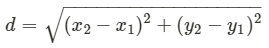The point that is exactly in the middle between two points is called the midpoint and is found by using one of the two following equations.
Method 1: For a number line with the coordinates a and b as endpoints: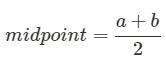Method 2: If we are working in a coordinate plane where the endpoints has the coordinates (x1,y1) and (x2,y2) then the midpoint coordinates is found by using the following formula: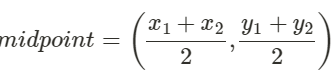Measure and classify an angle
A line that has one defined endpoint is called a ray and extends endlessly in one direction. A ray is named after the endpoint and another point on the ray e.g.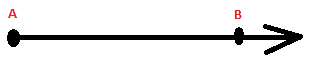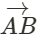The angle that is formed between two rays with the same endpoint is measured in degrees. The point is called the vertex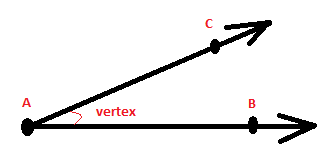The vertex is written as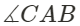In algebra we used the coordinate plane to graph and solve equations.  You can plot lines, line segments, rays and angles in a coordinate plane.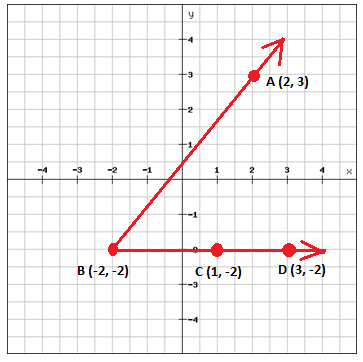In the coordinate plane above we have two rays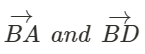That form an angle with the vertex in point B.
You can use the coordinate plane to measure the length of a line segment. Point B is at (-2, -2) and C (1. -2). The distance between the two points is 1 - (-2) = 3 units.
Angles can be either straight, right, acute or obtuse.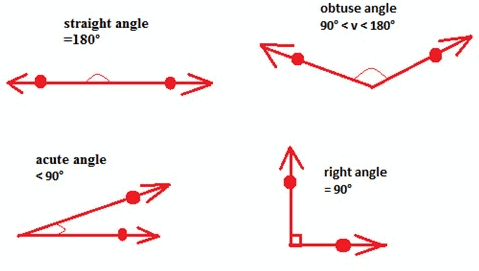An angle is a fraction of a circle where the whole circle is 360°. A straight angle is the same as half the circle and is 180° whereas a right angle is a quarter of a circle and is 90°.
You measure the size of an angle with a protractor.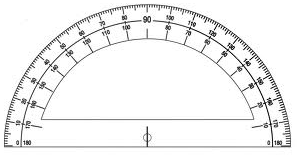Two angles with the same measure are called congruent angles. Congruent angles are denoted as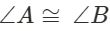Or could be shown by an arc on the figure to indicate which angles that are congruent.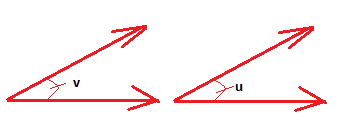Two angles whose measures together are 180° are called supplementary e.g. two right angles are supplementary since 90° + 90° = 180°.
Two angles whose measures together are 90° are called complementary.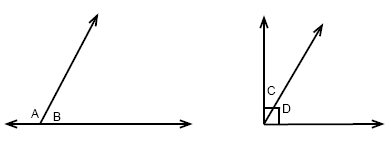m∠A  +m∠B = 180
m∠C + m∠D = 90

Polygons
A polygon is a closed figure where the sides are all line segments. Each side must intersect exactly two others sides but only at their endpoints. The sides must be noncollinear and have a common endpoint.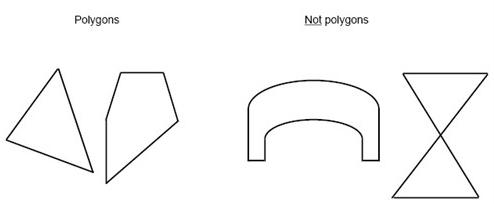A polygon is usually named after how many sides it has, a polygon with n-sides is called a n-gon. E.g. the building which houses United States Department of Defense is called pentagon since it has 5 sides.

 Sides Polygon 3 triangle 4 quadrilateral 5 pentagon 6 hexagon 7 heptagon 9 nonagon 10 decagon

A regular polygon is a polygon in which all sides are congruent and all the angles are congruent.

Offer running on EduRev: Apply code STAYHOME200 to get INR 200 off on our premium plan EduRev Infinity!

40 docs

,

,

,

,

,

,

,

,

,

,

,

,

,

,

,

,

,

,

,

,

,

,

,

,

,

,

,

;